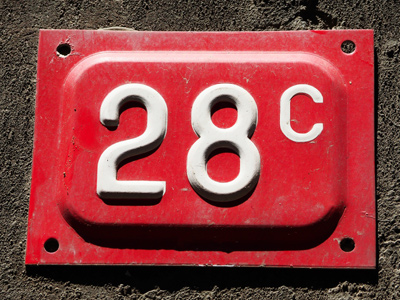7 × 4 = 28.

# 7 Times Table

Multiplying numbers by 7 is what learning the seven times table is about! You may wish to never see a times table again - but keep on practising until you know every answer automatically. Learning your times tables will be beneficial in the long run - you'll find multiplying numbers much easier and that'll help you in your 11-Plus.

The number 7 was considered a holy number by many. The world was said to have been created in seven days, that's why there are seven days in a week. There are seven deadly sins: avarice, envy, gluttony, lust, pride, sloth and wrath. Netball and water polo are both played with teams of seven players. Seven is a number you'll come across quite often.

The seven times table is one of the trickier ones so make sure you practise it a lot. Keep coming back to this quiz even after you think you've mastered it. The more often you test yourself the easier you'll find remembering your times tables.

1.
7 × 3 = ?
31
41
21
11
There are 21 spots on a six-sided die. That's because 1 + 2 + 3 + 4 + 5 + 6 = 21
2.
7 × 12 = ?
60
50
77
84
It takes Uranus 84 Earth years to orbit the Sun
3.
7 × 7 = ?
59
69
39
49
7 is the square root of 49
4.
7 × 9 = ?
36
63
53
43
70 - 7 = 63
5.
7 × 11 = ?
75
76
77
79
To times 7 by 11, first multiply 7 x 10 and then add 7
6.
7 × 4 = ?
28
38
48
18
The Danish, Swedish, Arabic and Esperanto alphabets all have 28 letters
7.
7 × 2 = ?
24
18
24
14
To double a number just add it to itself
8.
7 × 5 = ?
45
35
25
55
To multiply 7 by 5, first work out 7 x 10 and then halve your answer
9.
7 × 6 = ?
32
52
42
22
You can work this one out by multiplying 7 x 5 and then adding 7
10.
7 × 8 = ?
66
56
46
36
56 is the sum of the first 6 prime numbers. 3 + 5 + 7 + 11 + 13 + 17 = 56
Author:  Frank Evans